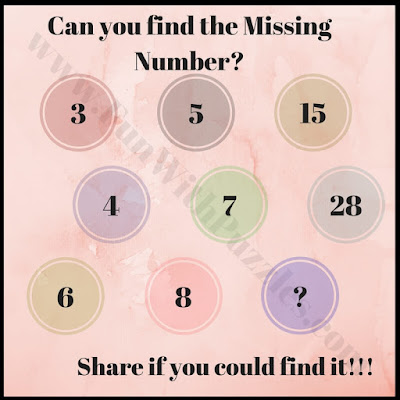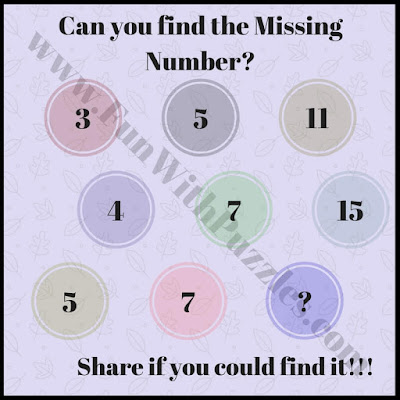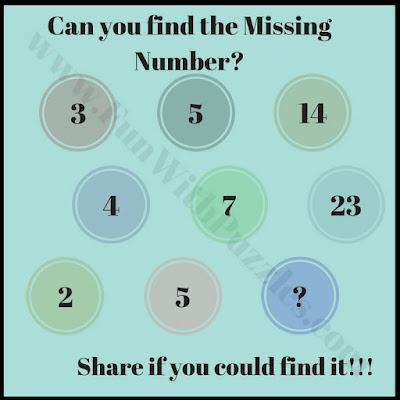There are many different types of Math Brain Teasers. In some of the Math Brain Teasers, some numbers will be given around a particular shape. These numbers share some mathematical pattern or sequence and one has to find the missing number while finding the mathematical pattern which connects these numbers. There are some equation math brain teasers in which one some mathematical equations are given with numbers being replaced with pictures and one has to find the value of these pictures while solving these maths equations. Some Math Brain Teasers are Number Puzzles in which one has to find the next number in the sequence. Here are some Math Number Sequence puzzles in which one has to find the missing number in the series.
In these Number Puzzles, 9 circles are given which each circle containing a number. 8 out of these 9 numbers are given and one number is missing. These numbers relate to each other mathematically. You challenge is to find this maths relationship among given numbers and then find the number which will replace the question mark.
Are you ready for this Number Puzzle Challenge? These brain teasers are arranged from easy to hard difficulty level. First few puzzles are very easy and then the last couple of brain teasers are tough. Solve it yourself without looking at the answers of these puzzles.
Answers of these puzzles are given at the end. Use these answers only to validate your solutions.1. Very easy math number puzzle2. Easy math sequence brain teaser3. Can you find the missing number puzzle?4. Tricky math sequence number puzzle5. Mind-bending math sequence puzzle

These Maths Number Sequence Puzzles are very much fun to solve. It also increases your Mathematical ability,  Logical Reasoning and Brain Power. Do checkout below mentioned puzzles to test further your Maths, Logic and Visual Skills

A1. 13

A2. 48

A3. 17

A4. 11

A5. 9